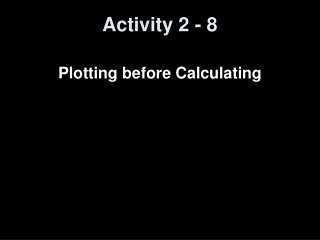DownloadDownload PresentationActivity 2 - 8

# Activity 2 - 8

Télécharger la présentation## Activity 2 - 8

- - - - - - - - - - - - - - - - - - - - - - - - - - - E N D - - - - - - - - - - - - - - - - - - - - - - - - - - -
##### Presentation Transcript

1. Activity 2 - 8 Plotting before Calculating

2. Objectives • Understand the need to first make a scatterplot of the date before calculating a correlation coefficient and regression line

3. Vocabulary • none new

4. Activity: 4 Data Sets • In 1973, statistician Frank Anscombe published the article “Graphs in Statistical Analysis” in the American Statistician, vol 27, pages 17-21. The article contained the following four sets of 11 pairs of data. • Instructions: Enter x-data for datasets 1, 2 and 3 into L1. Enter y-data for datasets 1, 2, 3 into L2, L3, and L4. Enter x-data for data set 4 into L5 and y-data into L6. Then run LinReg(ax+b) on each set of data and record your answers into the table below. Answer the questions below the table. Draw the scatterplots for each dataset on the graphs on the back. Also draw the regression lines on each graph. Answer to questions on the back.

5. Data Sets

6. Data Sets Scatterplots - 1 • Run a linear regression on the following data:

7. Data Sets Scatterplots - 2 • Run a linear regression on the following data:

8. Data Sets Scatterplots - 3 • Run a linear regression on the following data:

9. Data Sets Scatterplots - 4 • Run a linear regression on the following data:

10. Outlier Removed Scatterplots - 3 • Remove the outlier and run the regression again

11. So What’s the Point? • r is the linear correlation coefficient • The closer r is to 1, then the closer the data is to a positively sloped line • The closer r is to -1, then the closer the data is to a negatively sloped line • r can be deceiving • r values close to 1 or -1 can be seen without the graphs being linear • All equations are roughly y = 0.5 x + 3 and r-values are roughly 0.816

12. Summary and Homework • Summary • You can’t go by the r-value • You must always examine a graph of the data • Homework • Finish up the lab if you didn’t get it done in class